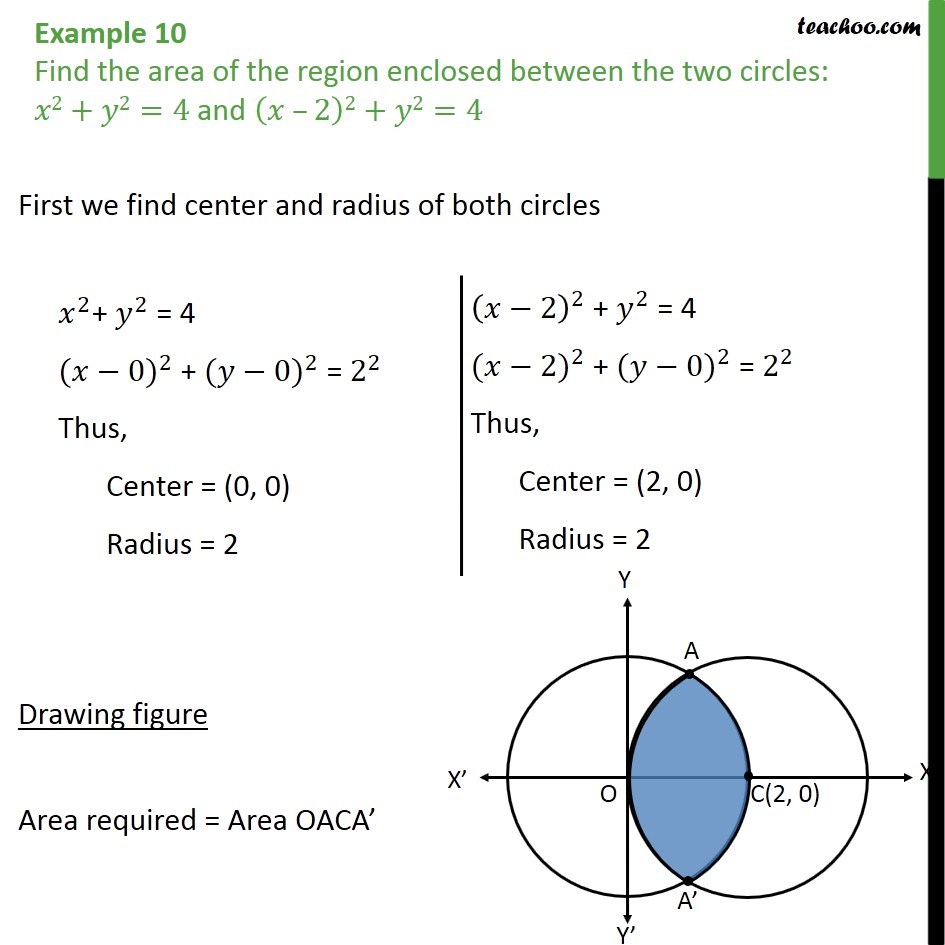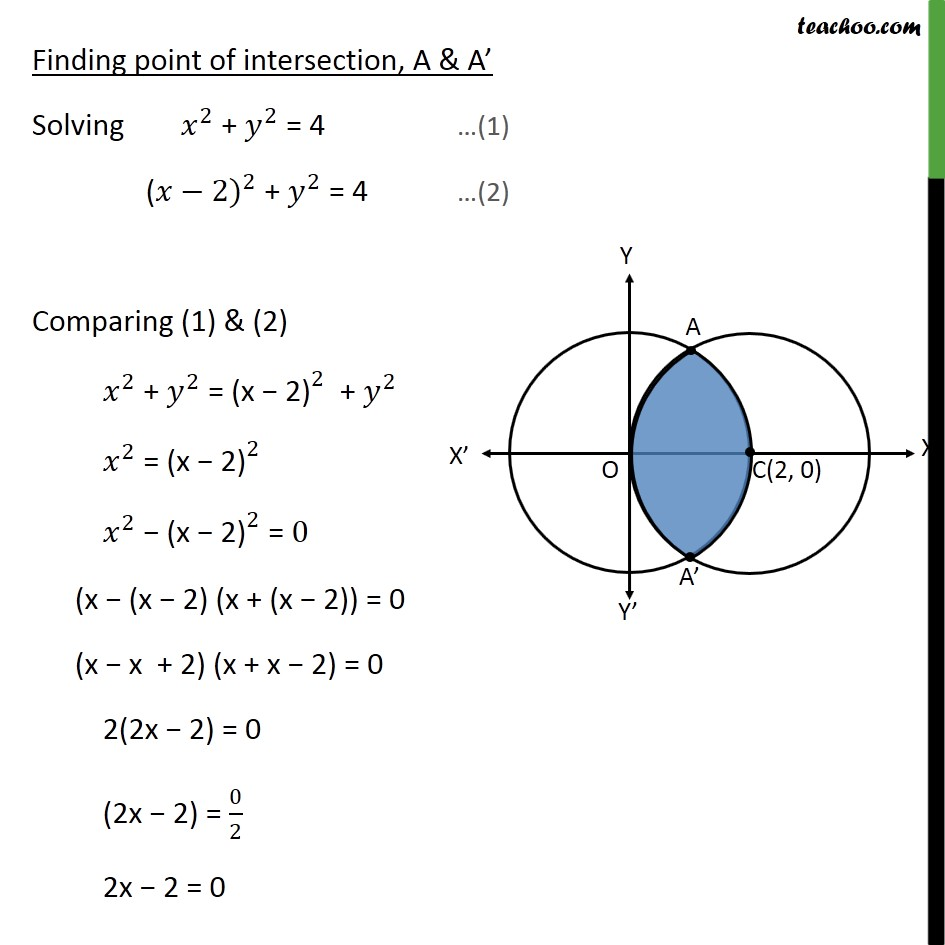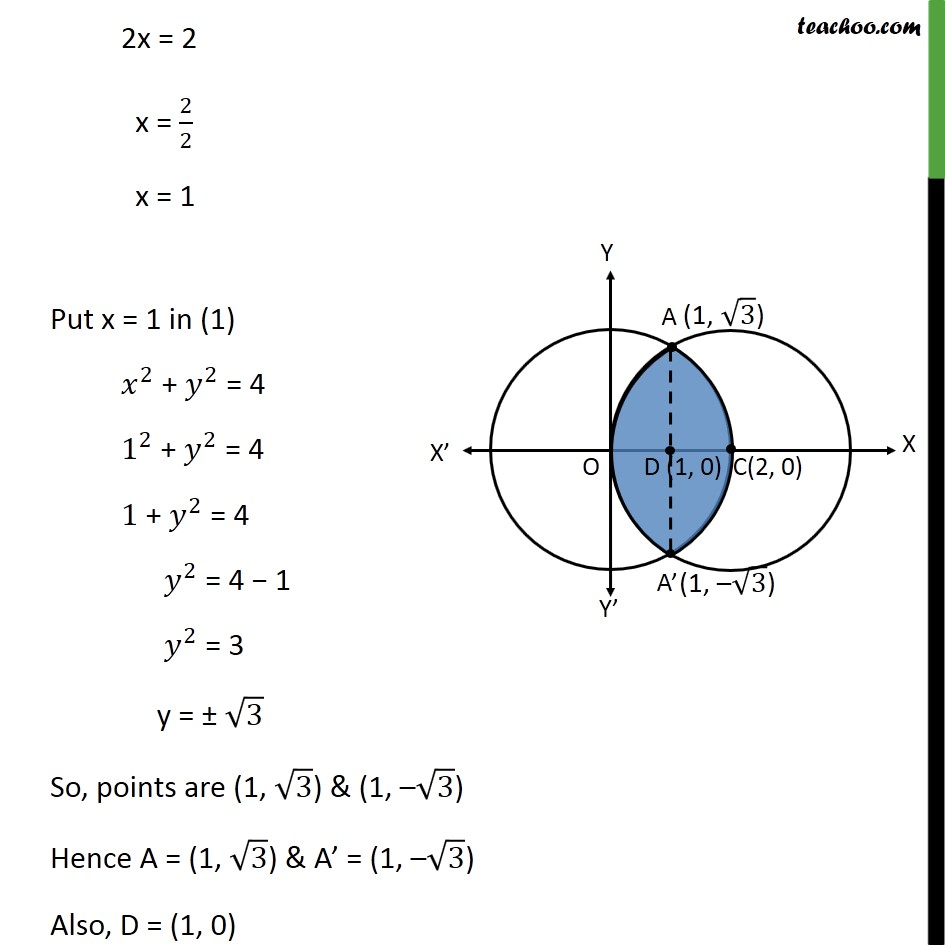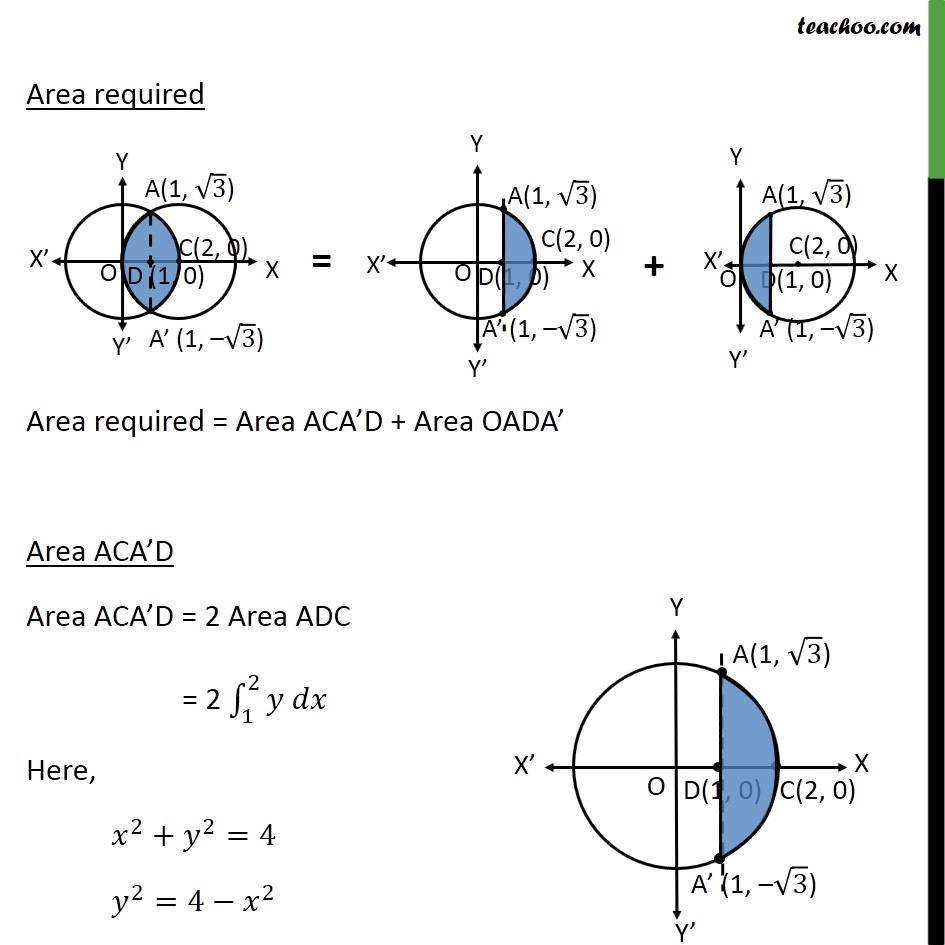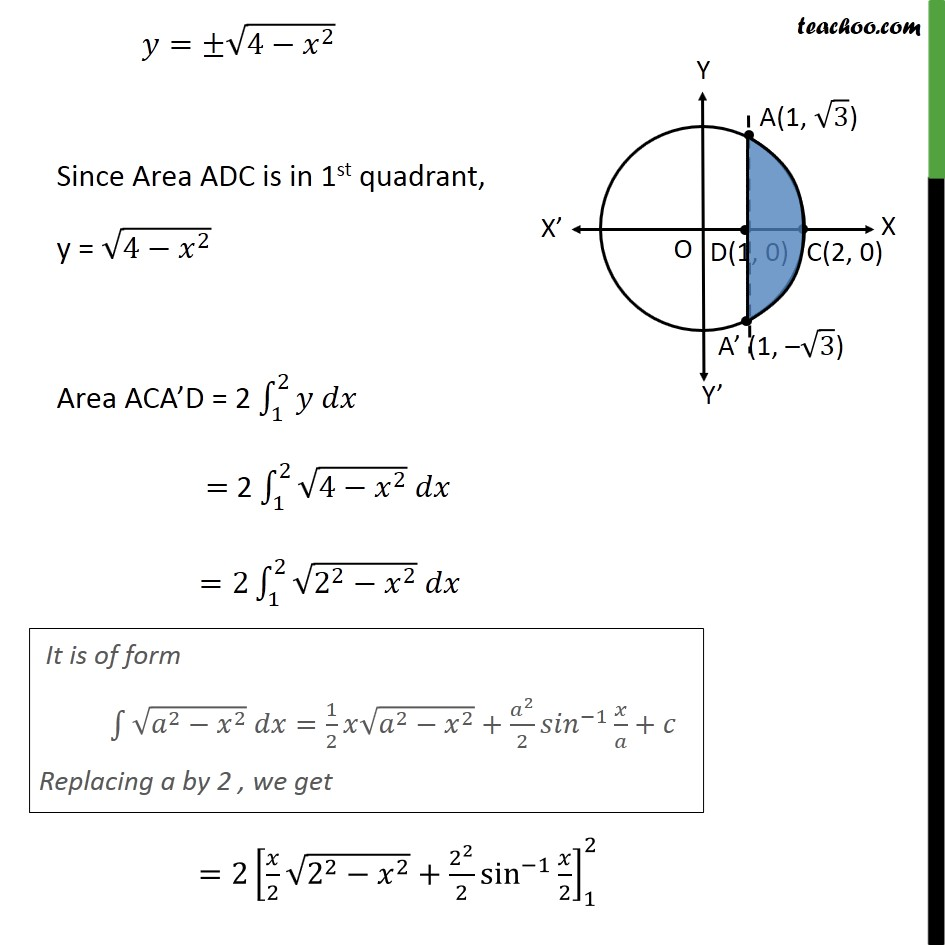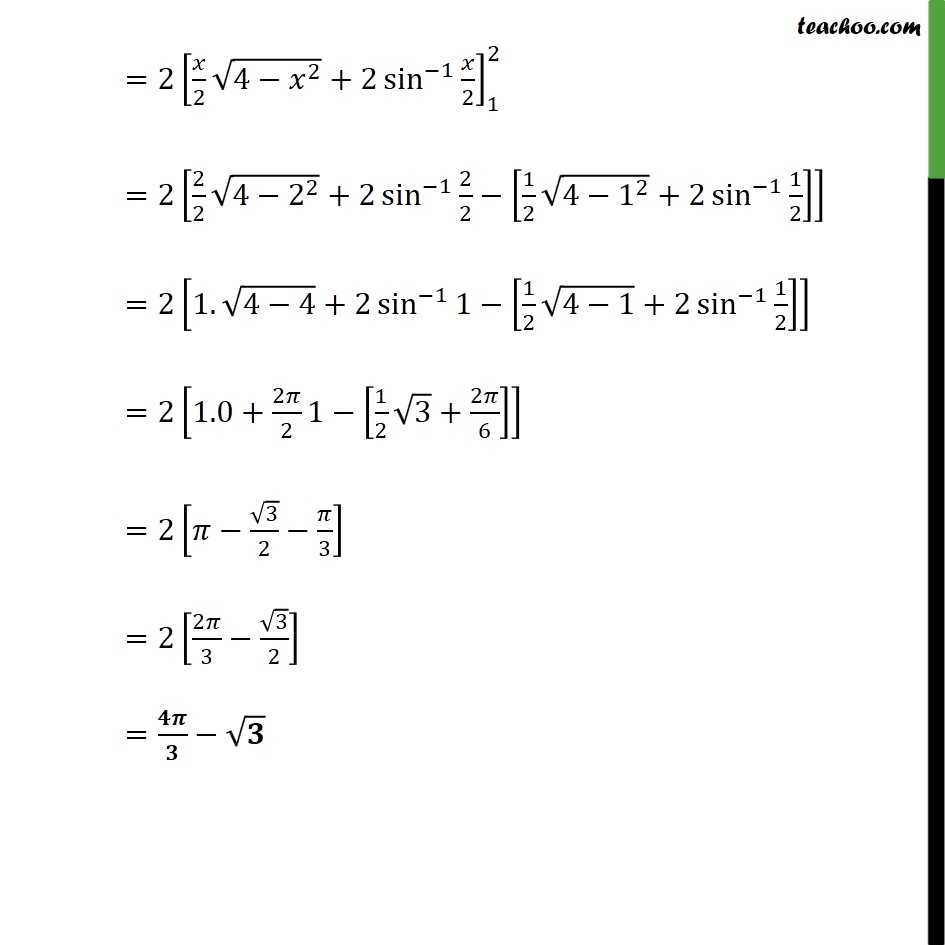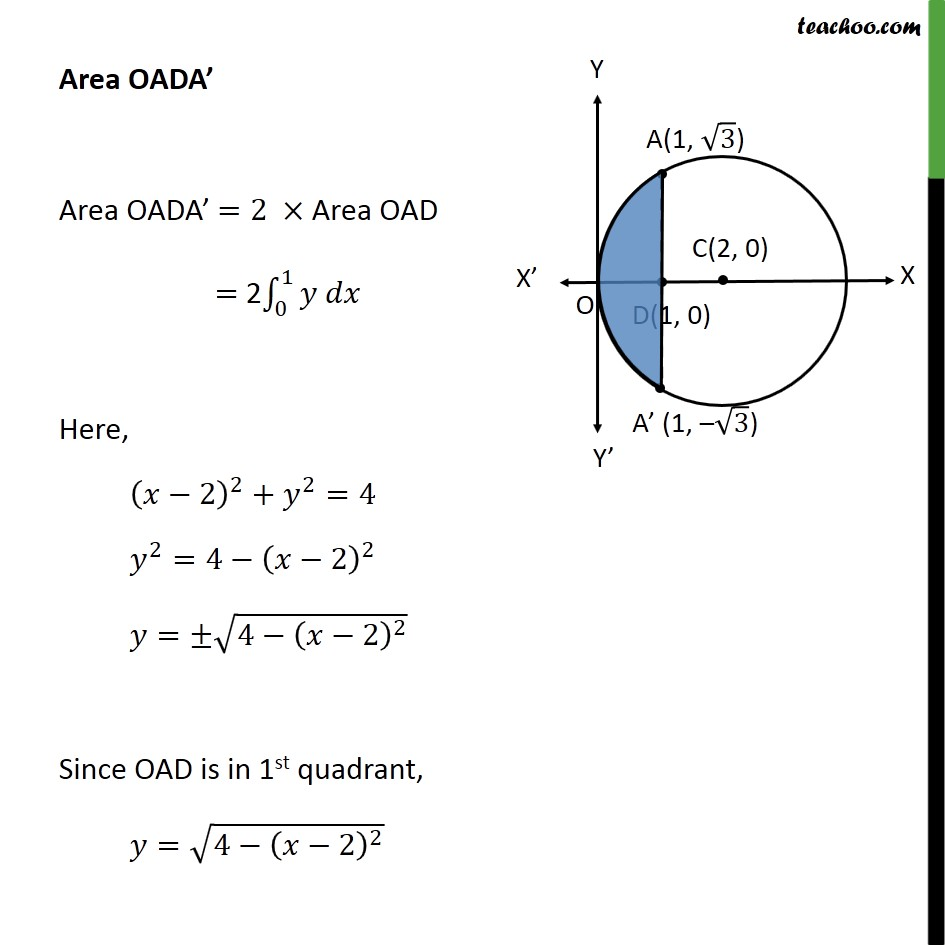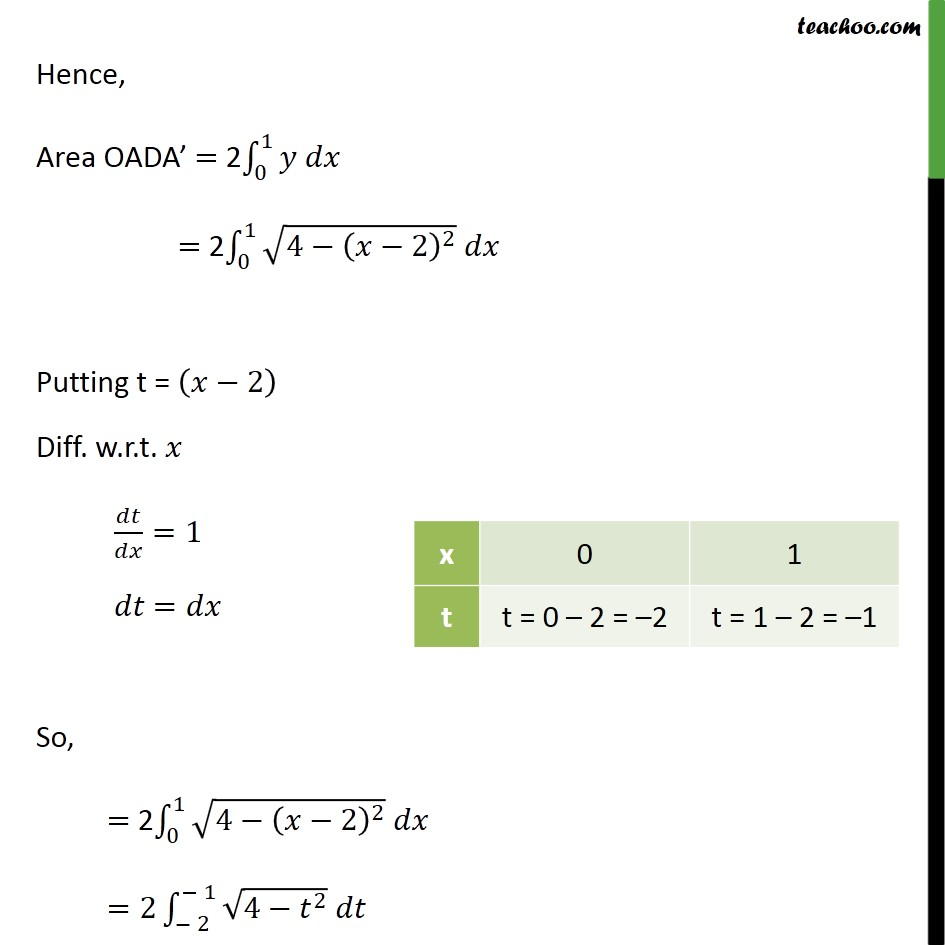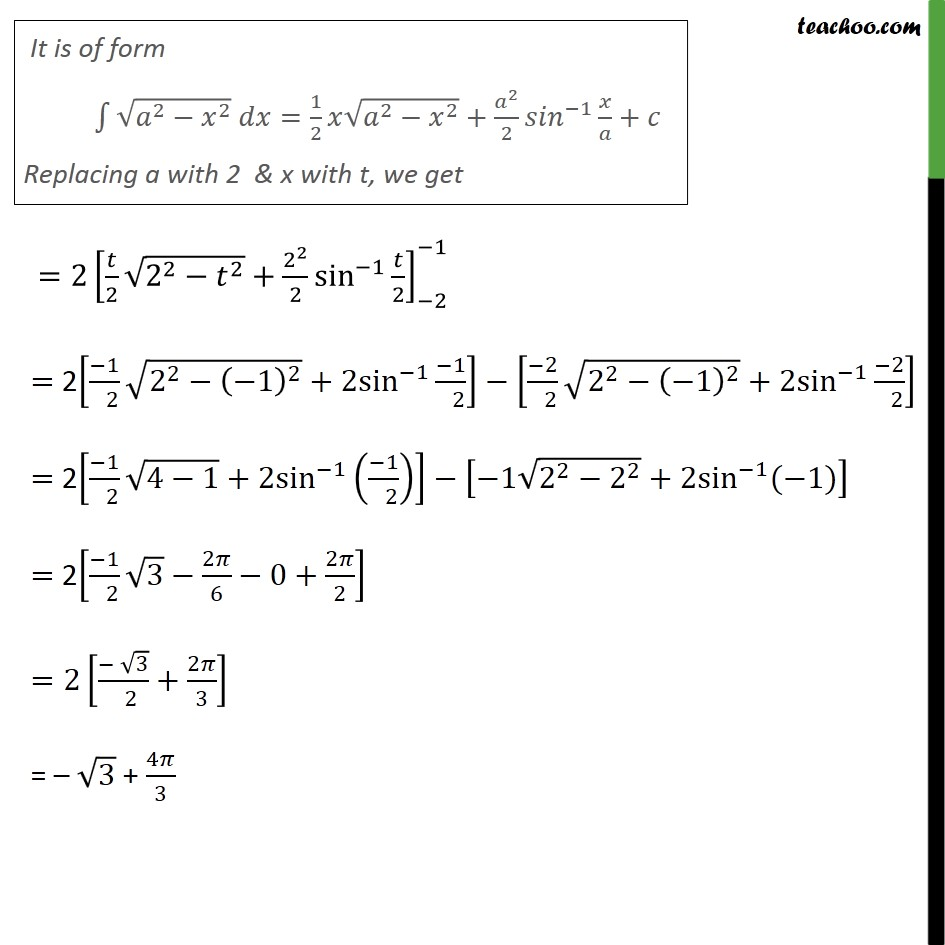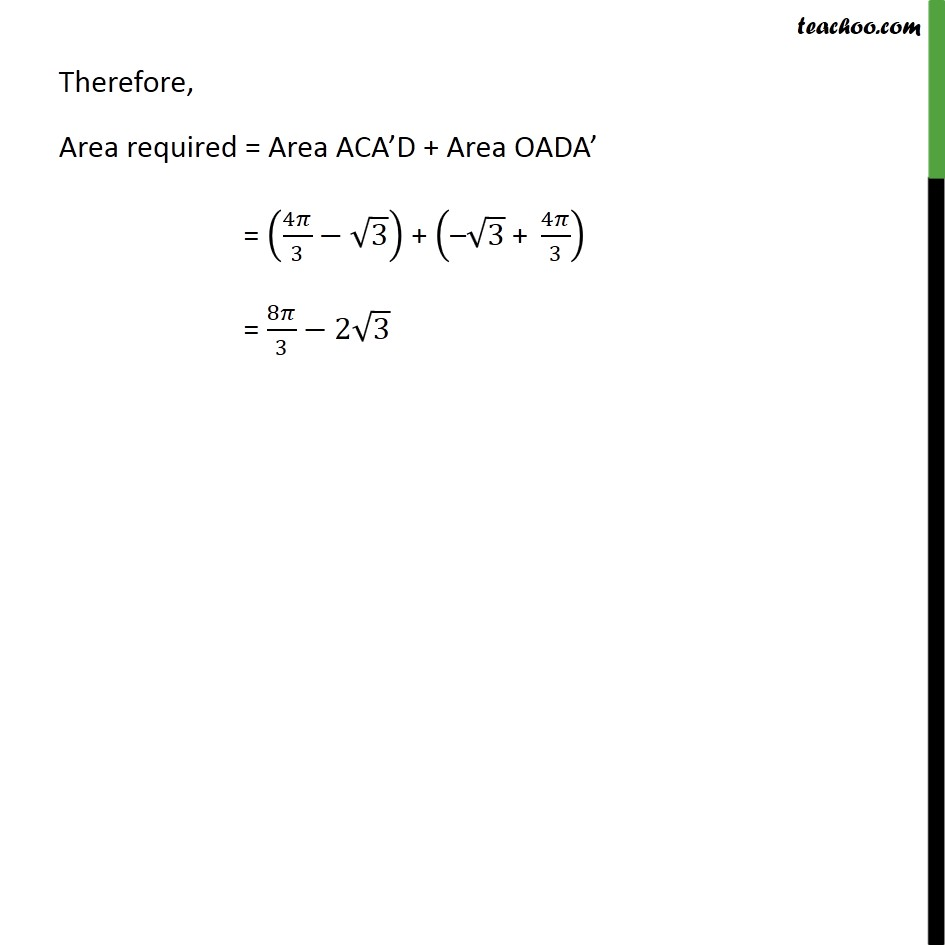1. Class 12
2. Important Question for exams Class 12
3. Chapter 8 Class 12 Application of Integrals

Transcript

Example 10 Find the area of the region enclosed between the two circles: 𝑥2+𝑦2=4 and 𝑥 –2﷯2+𝑦2=4 First we find center and radius of both circles Drawing figure Area required = Area OACA’ Area required = Area ACA’D + Area OADA’ Finding point of intersection, A & A’ Solving 𝑥﷮2﷯ + 𝑦﷮2﷯ = 4 …(1) ( 𝑥−2)﷮2﷯ + 𝑦﷮2﷯ = 4 …(2) Comparing (1) & (2) 𝑥﷮2﷯ + 𝑦﷮2﷯ = (x − 2)﷮2﷯ + 𝑦﷮2﷯ 𝑥﷮2﷯ = (x − 2)﷮2﷯ 𝑥﷮2﷯ − (x − 2)﷮2﷯ = 0 (x − (x − 2) (x + (x − 2)) = 0 (x − x + 2) (x + x − 2) = 0 2(2x − 2) = 0 (2x − 2) = 0﷮2﷯ 2x − 2 = 0 2x = 2 x = 2﷮2﷯ x = 1 Put x = 1 in (1) 𝑥﷮2﷯ + 𝑦﷮2﷯ = 4 1﷮2﷯ + 𝑦﷮2﷯ = 4 1 + 𝑦﷮2﷯ = 4 𝑦﷮2﷯ = 4 − 1 𝑦﷮2﷯ = 3 y = ± ﷮3﷯ So, points are (1, ﷮3﷯) & (1, – ﷮3﷯) Hence A = (1, ﷮3﷯) & A’ = (1, – ﷮3﷯) Also, D = (1, 0) Area required Area required = Area ACA’D + Area OADA’ Area ACA’D Area ACA’D = 2 Area ADC = 2 1﷮2﷮𝑦 𝑑𝑥﷯ Here, 𝑥﷮2﷯+ 𝑦﷮2﷯=4 𝑦﷮2﷯=4− 𝑥﷮2﷯ 𝑦=± ﷮4− 𝑥﷮2﷯﷯ Since Area ADC is in 1st quadrant, y = ﷮4− 𝑥﷮2﷯﷯ Area ACA’D = 2 1﷮2﷮𝑦 𝑑𝑥﷯ = 2 1﷮2﷮ ﷮4− 𝑥﷮2﷯﷯ 𝑑𝑥﷯ =2 1﷮2﷮ ﷮ 2﷮2﷯− 𝑥﷮2﷯﷯ 𝑑𝑥﷯ =2 𝑥﷮2﷯ ﷮ 2﷮2﷯− 𝑥﷮2﷯﷯+ 2﷮2﷯﷮2﷯ sin﷮−1﷯﷮ 𝑥﷮2﷯﷯﷯﷮1﷮2﷯ = 2 𝑥﷮2﷯ ﷮4− 𝑥﷮2﷯﷯+2 sin﷮−1﷯﷮ 𝑥﷮2﷯﷯﷯﷮1﷮2﷯ =2 2﷮2﷯ ﷮4− 2﷮2﷯﷯+2 sin﷮−1﷯﷮ 2﷮2﷯− 1﷮2﷯ ﷮4− 1﷮2﷯﷯+2 sin﷮−1﷯﷮ 1﷮2﷯﷯﷯﷯﷯ =2 1. ﷮4−4﷯+2 sin﷮−1﷯﷮1− 1﷮2﷯ ﷮4−1﷯+2 sin﷮−1﷯﷮ 1﷮2﷯﷯﷯﷯﷯ =2 1.0+ 2𝜋﷮2﷯﷮1− 1﷮2﷯ ﷮3﷯+ 2𝜋﷮6﷯﷯﷯﷯ =2 𝜋− ﷮3﷯﷮2﷯− 𝜋﷮3﷯﷯ =2 2𝜋﷮3﷯− ﷮3﷯﷮2﷯﷯ = 𝟒𝝅﷮𝟑﷯− ﷮𝟑﷯ Area OADA’ Area OADA’ =2 × Area OAD = 2 0﷮1﷮𝑦 𝑑𝑥﷯ Here, 𝑥−2﷯﷮2﷯+ 𝑦﷮2﷯=4 𝑦﷮2﷯=4− 𝑥−2﷯﷮2﷯ 𝑦=± ﷮4− 𝑥−2﷯﷮2﷯﷯ Since OAD is in 1st quadrant, 𝑦= ﷮4− 𝑥−2﷯﷮2﷯﷯ Hence, Area OADA’ = 2 0﷮1﷮𝑦 𝑑𝑥﷯ = 2 0﷮1﷮ ﷮4− 𝑥−2﷯﷮2﷯﷯ 𝑑𝑥﷯ Putting t = 𝑥−2﷯ Diff. w.r.t. 𝑥 𝑑𝑡﷮𝑑𝑥﷯=1 𝑑𝑡 =𝑑𝑥 So, = 2 0﷮1﷮ ﷮4− 𝑥−2﷯﷮2﷯﷯ 𝑑𝑥﷯ =2 − 2﷮− 1﷮ ﷮4− 𝑡﷮2﷯﷯ 𝑑𝑡﷯ =2 𝑡﷮2﷯ ﷮ 2﷮2﷯− 𝑡﷮2﷯﷯+ 2﷮2﷯﷮2﷯ sin﷮−1﷯﷮ 𝑡﷮2﷯﷯﷯﷮−2﷮−1﷯ = 2 −1﷮ 2﷯ ﷮ 2﷮2﷯− −1﷯﷮2﷯﷯+2 sin﷮−1﷯ −1﷮ 2﷯﷯− −2﷮ 2﷯ ﷮ 2﷮2﷯− −1﷯﷮2﷯﷯+2 sin﷮−1﷯ −2﷮ 2﷯﷯ = 2 −1﷮ 2﷯ ﷮4−1﷯+2 sin﷮−1﷯ −1﷮ 2﷯﷯﷯− −1 ﷮ 2﷮2﷯− 2﷮2﷯﷯+2 sin﷮−1﷯(−1)﷯ = 2 −1﷮ 2﷯ ﷮3﷯− 2𝜋﷮6﷯−0+ 2𝜋﷮2﷯﷯ =2 − ﷮3﷯﷮ 2﷯+ 2𝜋﷮3﷯﷯ = – ﷮3﷯ + 4𝜋﷮3﷯ Therefore, Area required = Area ACA’D + Area OADA’ = 4𝜋﷮3﷯− ﷮3﷯﷯ + – ﷮3﷯ + 4𝜋﷮3﷯﷯ = 8𝜋﷮3﷯−2 ﷮3﷯

Chapter 8 Class 12 Application of Integrals

Class 12
Important Question for exams Class 12